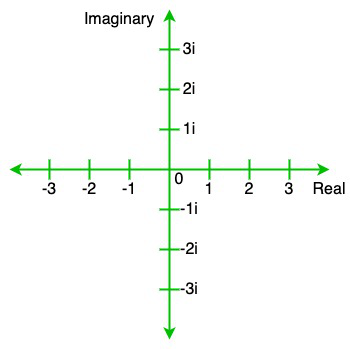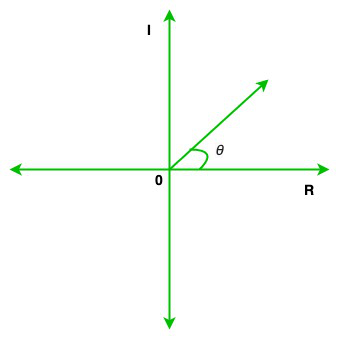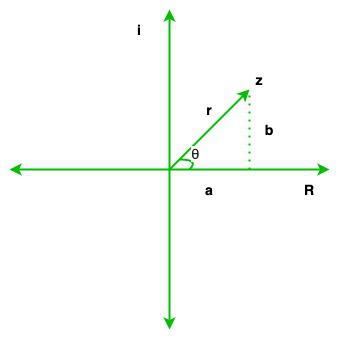# Absolute Value of a Complex Number

• Last Updated : 06 Jun, 2021

The complex number is defined as the number in the form a+ib, where a is the real part while ib is the imaginary part of the complex number in which i is known as iota and b is a real number. The value of i is √(-1). Or in other words, a complex number is a combination of real and imaginary numbers. For example, 5+11i, 10+20i, etc.

• Real number: A real number is a number that is present in the number system which can be positive, negative, integer, rational irrational, etc. For example, 23, -3, 3/6.
• Imaginary number: Imaginary numbers are those numbers that are not real numbers. For example, √3, √11, etc.
• Zero complex number: A zero complex number is a number that has its real and imaginary parts both equal to zero. For example, 0+0i.

Graphical representation of complex number:

The graphical representation of the complex number is as shown in the below image:Here, the real part of the complex number is represented on the horizontal axis while the imaginary part of the complex number is represented on the vertical axis.

### Absolute value

The absolute value(Modulus) of a number is the distance of the number from zero. Absolute value is always represented in the modulus(|z|) and its value is always positive. So, the absolute value of the complex number Z = a + ib is

|z| = √ (a2 + b2)

So, the absolute value of the complex number is the positive square root of the sum of the square of real part and the square of the imaginary part, i.e.,

Proof:

Let us consider the mode of the complex number z is extended from 0 to z and the mod of a, b real numbers is extended from a to 0 and b to 0. So these values create a right angle triangle in which 0 is the vertex of the acute angle

So, using Pythagoras theorem, we get,

|z|2 = |a|2 + |b|2

|z| = √ a2 + b2

Now, in the sets of complex numbers z1 > z2 or z1 < z2 has no meaning but |z1| > |z2| or |z1| < |z2| has meaning because |z1| and |z2| is a real number.

Properties of modulus of a complex number:

1. |z| = 0 <=> z = 0i, i.e., Re(z) = 0 and Im(z) = 0
2. |z| = || = |-z|
3. -|z| ≤ Re(z) ≤ |z|, -|z| ≤ Im(z) ≤ |z|
4. z.= |z2|
5. |z1z2| = |z1||z2|
6. |z1 / z2| = |z1|/|z2|
7. |z1 + z2|2 = |z1| + |z2| + 2Re(z1)
8. |z1 – z2|2 = |z1| + |z2| – 2Re(z1)
9. |z1 + z2|2 ≤ |z1| + |z2|
10. |z1 – z2|2 ≥ |z1| – |z2|
11. |az1 – bz2|2 + |bz1 + az2|2 = (a2 + b2)(|z1|2 + |z2|2) Or |z1 – z2|2 + |z1 + z2|2 = 2(|z1|2 + |z2|2)
12. |zn| = |z|n
13. 1/z = a – ib/a2 + b2 =/|z|2

Example:

(i) z = 3 + 4i

|z| = √(32 + 42)

= √(9 + 16)

= √25

= 5

(ii) z = 5 + 6i

|z| = √(52 + 62)

= √(25 + 36)

= √61

### Angle of Complex numbers

The angle of a complex number or argument of the complex number is the angle inclined from the real axis in the direction of the complex number that represents on the complex plane or argand plan.

θ = tan-1(b/a)

or

arg(Z) = tan-1(b/a)

Here, Z = a + ibProperties of the angel or argument of a complex number:

• arg(Zn) = n arg(Z)
• arg (Z1/ Z2) = arg (Z1) – arg (Z2)
• arg (Z1 Z2) = arg (Z1) + arg (Z2)

Examples:

(i) z = 2 + 2i

θ = tan-1(2/2)

= tan-1(1)

= 45°

(ii) z = -4 + 4i

θ = tan-1(4/-4)

= tan-1(-1)

= -45°

It is important to note here that  the angle θ =-45° is in 4th quadrant,

while we always measure angle with the positive x-axis.

So, we will have to add 180° to the answer to obtain the real  opposite angle.

So, θ = 180° + (-45°)

= 135°

So , the above complex number will make an angle of 135° with the positive x-axis.

### Polar Form of Complex number

The polar form of complex number is also a way to represent a complex number. Generally, we represent complex number like Z = a + ib, but in polar form, complex number is represented in the combination of modulus and argument. Here, the modulus of the complex number is known as the absolute value of the complex number, and the argument is known as the angle of the complex number.

Z = r(cos θ + isin θ)

Proof:

Let us considered we have a complex number Z = a+ib. So we draw an argand plane, it is a plane where we can represent complex numbers it is also known as a complex plan. In the argand plan, the horizontal line represents the real axis and the vertical line represents the imaginary axis. Now we plot a on the real axis and b on the imaginary axis. Now we represent vector Z as a position vector that starts at 0 and the tip is at coordinate (a, b). Suppose θ be the angle made by Z over the real axis and the distance between 0 to Z is r(it is also known as the magnitude/absolute value of vector Z). Here, (r, θ) pair is known as the polar coordinates of Z.According to the diagram, we have a right angle triangle

So, using Pythagoras’s theorem, we get,

r = |z|2 = |a|2 + |b|2

r = |z| = √ a2 + b2

This is the modulus

Now we find the value of θ

tan θ = (a/b)

θ = tan-1(a/b)

This is the argument

Now we find the polar form of the complex number:

So using the trigonometric formula, we get

cos θ = a/r

Now multiply both sides with r we get

rcos θ = a

sin θ = b/r

Now multiply both sides with r we get

rsin θ = b

So, we get

Z = rcos θ + irsin θ

Z = r(cos θ + isin θ)

Here, r is the absolute value of the complex number and θ is the argument of the complex number.

The exponential form of complex numbers:

The exponential form of complex numbers uses both the trigonometric ratios of sine and cosine to define the complex exponential as a rotating plane in exponential form. The exponential form of a complex number is generally given by Euler’s Identity, named after famous mathematician Leonhard Euler. It is given as follows:

Z = re

Example:

(i) Given that r = 5 and  θ = 45°. Find the polar form of the complex number

z = rcosA + (rsinA)i

z = 5cos45° + (5sin45°)i

= 5(1/√2) + (5 (1/√2))i

= 5/√2 + (5/√2)i

(ii) Given that r = 6 and  θ = 30°. Find the polar form of the complex number

z = rcosA + (rsinA)i

z = 6cos30° + (6sin30°)i

= 6(√3/2) + (6(1/2))i

= 3√3 + 3i

Convert rectangular form to polar form:

Let us discuss this concept with the help of an example:

Suppose we have a complex number, i.e., Z = 3 + 4i

It is in rectangular form so now we have to convert it to a polar form.

Step 1: So, first, we will calculate the modulus of the complex numbers and then the angle.

|Z|= √(32 + 42)

= √(9 + 16)

= √25

= 5

Step 2: Now we find the angle of the complex number,

θ  = tan-1(y/x)

= tan-1(4/3)

= 53.1°

Step 3: As we know that the formula of polar form is:

Z = r(cos θ + isin θ)

Now put the value of r and θ in this equation, we get

Z = 5(cos 53.1 + isin 53.1)

Hence, the polar from of 3 + 4i is 5(cos 53.1 + isin 53.1)

### Sample Problems

Question 1. Find the absolute value of z = 4 + 8i

Solution:

Given complex number is z = 4 + 8i

As we know that the formula of absolute value is

|z| = √ (a2 + b2)

So, a = 4, and b = 8, we get

|z| = √(42 + 82)

|z| = √80

Question 2. Find the absolute value of z = 2 + 4i

Solution:

Given complex number is z = 2 + 4i

As we know that the formula of absolute value is

|z| = √ (a2 + b2)

So, a = 2, and b = 4, we get

|z| = √(22 + 42)

|z| = √20

Question 3. Find the angle of the complex number: z = √3 + i

Solution:

Given complex number is z = √3 + i

As we know, that

θ = tan-1(b/a)

So, a = √3 , and b = 1, we get

θ = tan-1(1/ √3 )

θ = 30°

Question 4. Find the angle of the complex number: z = 6 + 6i

Solution:

Given complex number is z = 6 + 6i

As we know, that

θ = tan-1(b/a)

So, a = 6 , and b = 6, we get

θ = tan-1(6/6)

θ = 45°

Question 5. Convert z =  5 + 5i into polar form

Solution:

Given complex number is z = 5 + 5i

As we know that

Z = r(cos θ + isin θ) …(1)

Now, we find the value of r

r = √(52 + 52)

r = √(25 + 25)

r = √50

Now we find the value of θ

θ = tan-1(5/5)

θ = tan-1(1)

θ = 45°

Now put all these values in eq(1), we get

Z = √50(cos 45° + isin 45°)

Question 6. Convert z =  2 + √3i into polar form

Solution:

Given complex number is z = 2 + 2√3i

As we know that

Z = r(cos θ + isin θ) …(1)

Now, we find the value of r

r = √(22 + (2√3)2)

r = √(4 + 12)

r = √16

r = 4

Now we find the value of θ

θ = tan-1(2√3/2)

θ = tan-1(√3)

θ = 60°

Now put all these values in eq(1), we get

Z = 4(cos 60° + isin 60°)

My Personal Notes arrow_drop_up
Recommended Articles
Page :